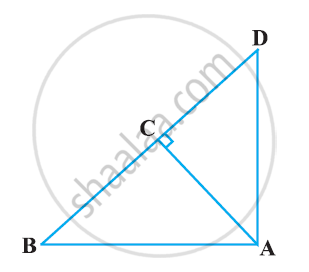Share

# In Figure, ABD is a triangle right angled at A and AC ⊥ BD. Show that AB^2 = BC × BD - CBSE Class 10 - Mathematics

#### Question

In Figure, ABD is a triangle right angled at A and AC ⊥ BD. Show that AB2 = BC × BD#### Solution

In ΔADB and ΔCAB, we have

∠DAB = ∠ACB (Each equals to 90°)

∠ABD = ∠CBA (Common angle)

∴ ΔADB ~ ΔCAB [AA similarity criterion]

⇒(AB)/(CB) = (BD)/(AB)

⇒ AB2 = CB × BD

Is there an error in this question or solution?

#### APPEARS IN

NCERT Solution for Mathematics Textbook for Class 10 (2019 to Current)
Chapter 6: Triangles
Ex. 6.50 | Q: 3.1 | Page no. 150

#### Video TutorialsVIEW ALL 

Solution In Figure, ABD is a triangle right angled at A and AC ⊥ BD. Show that AB^2 = BC × BD Concept: Pythagoras Theorem.
S